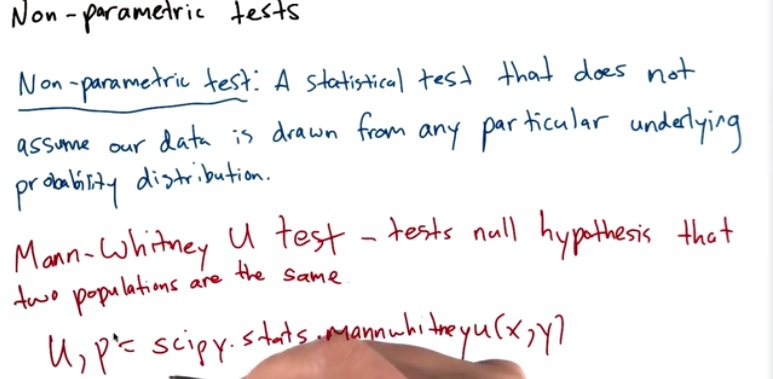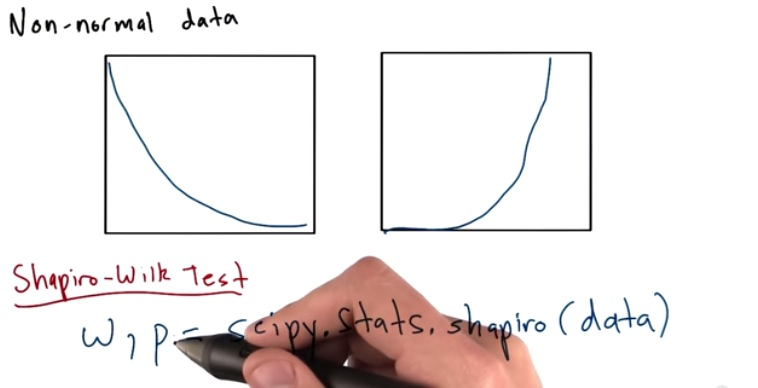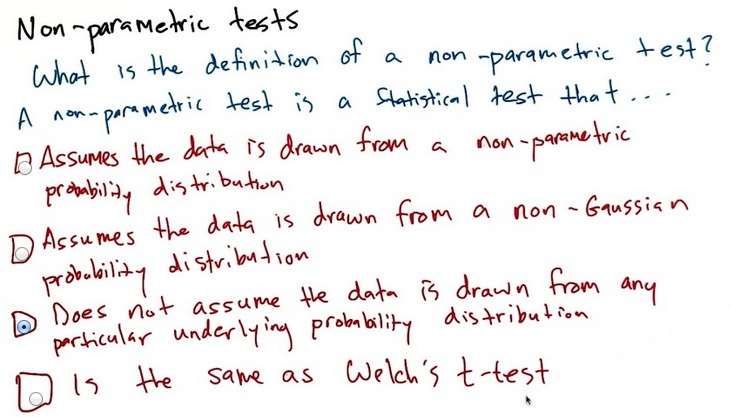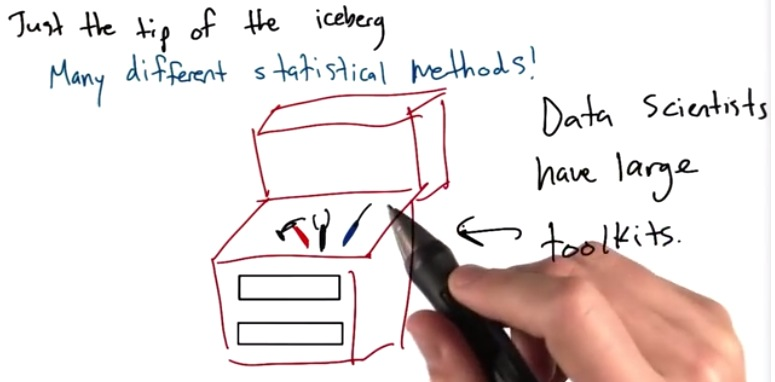• non-parametric test assume that both are not from the same population(probability distribution), but still want to test the similarity between them(null hypothesis). Or it want to test wheter two population come from the same population
• then we can make non-parametric test(eventhough t-test is also welcome).
• More useful if the calculations is also returning median and mean. compare them• Sometimes we have non-normal data, that we think it's similar
• We want to know if both samples above are the same gaussian distribution
• Make a histogram and plot if both have the same curve(which as we can see, no)
• We can make both the same
• w value is denotes from shapiro-wilk value test
• p is like p in t-test, which determines the likelihood the w is as extreme as given our null hypothesis is the same• non-parametric is a term given statistical test, and not used for probability distribution
• It also doesn't assume point c, as oppose to welch's t-test, which assume point c• There's still many statistical test that data scientist used which we just know until know like the tip of an iceberg.
• It's important to know all these so we can use tests suitable to the data that we have.